## a particle covers one fourth of its distance with speed v and remaining three fourth with speed 2v. the average speed of particle is:1.8/5v,

Question

a particle covers one fourth of its distance with speed v and remaining three fourth with speed 2v. the average speed of particle is:1.8/5v,2.3/2v,3.4/3v,4.7/5v

in progress 0
6 months 2021-07-17T12:29:27+00:00 2 Answers 3 views 0

1. Answer: 1.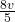Explanation: Speed is a variable in Physics defined by the rate at which an object go through a distance. It can be calculated as: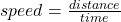A fourth of distance with speed v takes: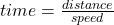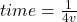The rest of the distance with speed 2v takes: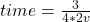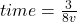Average speed is given by: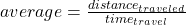Total time to cover the distance: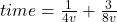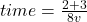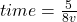Total distance traveled: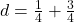d = 1

average = 1 ÷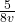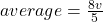The average speed of particle is 8/5v.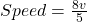Explanation:

Given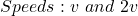Required

Determine the average speed

First, we need to determine the time spent on the journey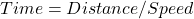For the first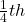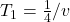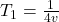For the next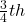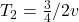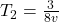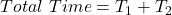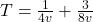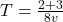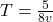Average Speed is then calculated as thus: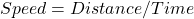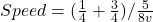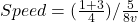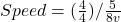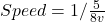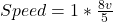Hence;